# 5.4.5 - Data Quality Reports - Teradata Warehouse Miner

## Teradata Warehouse Miner User Guide - Volume 3Analytic Functions

Product
Release Number
5.4.5
Published
February 2018
Language
English (United States)
Last Update
2018-05-04
dita:mapPath
yuy1504291362546.ditamap
dita:ditavalPath
ft:empty
dita:id
tbj1499730075274

## Variable Statistics

If selected on the Results Options tab, this report gives the mean value and standard deviation of each variable in the model based on the SSCP matrix provided as input.

## Near Dependency

If selected on the Results Options tab, this report lists collinear variables or near dependencies in the data based on the SSCP matrix provided as input. Entries in the Near Dependency report are triggered by two conditions occurring simultaneously. The first is the occurrence of a large condition index value associated with a specially constructed principal factor. If a factor has a condition index greater than the parameter specified on the Results Option tab, it is a candidate for the Near Dependency report. The other is when two or more variables have a variance proportion greater than a threshold value for a factor with a high condition index. Another way of saying this is that a ‘suspect’ factor accounts for a high proportion of the variance of two or more variables. The parameter to defines what a high proportion of variance is also set on the Results Option tab. A default value of 0.5.

## Detailed Collinearity Diagnostics

If selected on the Results Options tab, this report provides the details behind the Near Dependency report, consisting of the following tables.
• Eigenvalues of Unit Scaled X'X — Report of the eigenvalues of all variables scaled so that each variable adds up to 1 when summed over all the observations or rows. In order to calculate the singular values of X (the rows of X are the observations), the mathematically equivalent square root of the eigenvalues of XTX are computed instead for practical reasons.
• Condition Indices — The condition index of each eigenvalue, calculated as the square root of the ratio of the largest eigenvalue to the given eigenvalue, a value always 1 or greater.
• Variance Proportions — The variance decomposition of these eigenvalues is computed using the eigenvalues together with the eigenvectors associated with them. The result is a matrix giving, for each variable, the proportion of variance associated with each eigenvalue.

## Logistic Regression Step N (Stepwise-only)

• In Report — This report is the same as the Variables in Model report, but it is provided for each step during stepwise logistic regression based on the variables currently in the model at each step.
• Out Report
• Column Name — The independent variable excluded from the model.
• W Statistic — The W Statistic is a specialized statistic designed to determine the best variable to add to a model without calculating a maximum likelihood solution for each variable outside the model. The W statistic is assumed to follow a chi square distribution with one degree of freedom due to its similarity to other statistics, and it gives evidence of behaving similarly to the likelihood ratio statistic. For more information, refer to [Peduzzi, Hardy and Holford].
• Chi Sqr P-value — The W statistic is assumed to follow a chi square distribution on one degree of freedom due to its similarity to other statistics, and it gives evidence of behaving similarly to the likelihood ratio statistic. Therefore, the variable with the smallest chi square probability or P-value associated with its W statistic is added to the model in a forward step if the P-value is less than the criterion to enter.

## Logistic Regression Model

• Total Observations — This is the number of rows in the table that the logistic regression analysis is based on. The number of observations reflects the row count after any rows were eliminated by listwise deletion (due to one of the variables being null).
• Total Iterations — The number of iterations used by the non-linear optimization algorithm in maximizing the log likelihood function.
• Initial Log Likelihood — The initial log likelihood is the log likelihood of the constant only model and is given only when the constant is included in the model. The formula for initial log likelihood is given by: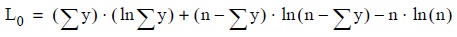where n is the number of observations.

• Final Log Likelihood — This is the value of the log likelihood function after the last iteration.
• Likelihood Ratio Test G Statistic — Deviance, given by D = -2L M , where L M is the log likelihood of the logistic regression model, is a measure analogous to the residual sums of squares RSS in a linear regression model. In order to assess the utility of the independent terms taken as a whole in the logistic regression model, the deviance difference statistic G is calculated for the model with a constant term only versus the model with all variables fitted. This statistic is then G = -2(L 0 - L M ), where L 0 is the log likelihood of a model containing only a constant. The G statistic, like the deviance D, is an example of a likelihood ratio test statistic.
• Chi-Square Degrees of Freedom — The G Statistic follows a chi-square distribution with “variables minus one” degrees of freedom. This field then is the degrees of freedom for the G Statistic’s chi-square test.
• Chi-Square Value — This is the chi-square random variable value for the Likelihood Ratio Test G Statistic. This can be used to test whether all the independent variable coefficients should be 0. Examining the field Chi-square Probability is however the easiest way to assess this test.
• Chi-Square Probability — This is the chi-square probability value for the Likelihood Ratio Test G Statistic. It can be used to test whether all the independent variable coefficients should be 0. That is, the probability that a chi-square distributed variable would have the value G or greater is the probability associated with having all 0 coefficients. The null hypothesis that all the terms should be 0 can be rejected if this probability is sufficiently small, say less than 0.05.
• McFadden's Pseudo R-Squared — To mimic the Squared Multiple Correlation Coefficient (R 2 ) in a linear regression model, the researcher McFadden suggested this measure given by (L 0 - L M ) / L 0 where L 0 is the log likelihood of a model containing only a constant and L M is the log likelihood of the logistic regression model. Although it is not truly speaking a goodness of fit measure, it can be useful in assessing a logistic regression model. Experience shows that the value of this statistic tends to be less than the R 2 value it mimics. In fact, values between 0.20 and 0.40 are quite satisfactory.
• Dependent Variable Name — Column chosen as the dependent variable.
• Dependent Variable Response Values — The response value chosen for the dependent variable on the Regression Options tab.
• Dependent Variable Distinct Values — The number of distinct values that the dependent variable takes on.

## Logistic Regression Variables in Model report

• Column Name — This is the name of the independent variable in the model or CONSTANT for the constant term.
• B Coefficient — The b-coefficient is the coefficient in the logistic regression model for this variable. The following equations describe the logistic regression model, with being the probability that the dependent variable is 1, and g(x) being the logit transformation: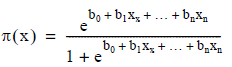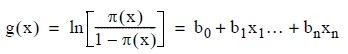• Standard Error — The standard error of a b-coefficient in the logistic regression model is a measure of its expected accuracy. It is analogous to the standard error of a coefficient in a linear regression model.
• Wald Statistic — The Wald statistic is calculated as the square of the T-statistic (T Stat) described below. The T-statistic is calculated for each b-coefficient as the ratio of the b-coefficient value to its standard error.
• T Statistic — In a manner analogous to linear regression, the T-statistic is calculated for each b-coefficient as the ratio of the b-coefficient value to its standard error. Along with its associated t-distribution probability value, it can be used to assess the statistical significance of this term in the model.
• P-value — This is the t-distribution probability value associated with the T-statistic (T Stat), that is, the ratio of the b-coefficient value (B Coef) to its standard error (Std Error). It can be used to assess the statistical significance of this term in the logistic regression model. A value close to 0 implies statistical significance and means this term in the model is important.

The P-value represents the probability that the null hypothesis is true, that is the observation of the estimated coefficient value is chance occurrence (i.e., the null hypothesis is that the coefficient equals zero). The smaller the P-value, the stronger the evidence for rejecting the null hypothesis that the coefficient is actually equal to zero. In other words, the smaller the P-value, the larger the evidence that the coefficient is different from zero.

• Odds Ratio — The odds ratio for an independent variable in the model is calculated by taking the exponent of the b-coefficient. The odds ratio is the factor by which the odds of the dependent variable being 1 change due to a unit increase in this independent variable.
• Lower — Because of the intuitive meaning of the odds ratio, confidence intervals for coefficients in the model are calculated on odds ratios rather than on the coefficients themselves. The confidence interval is computed based on a 95% confidence level and a two-tailed normal distribution. “Lower” is the lower range of this confidence interval.
• Upper — Because of the intuitive meaning of the odds ratio, confidence intervals for coefficients in the model are calculated on odds ratios rather than on the coefficients themselves. The confidence interval is computed based on a 95% confidence level and a two-tailed normal distribution. “Upper” is the upper range of this confidence interval.
• Partial R — The Partial R statistic is calculated for each b- coefficient value as: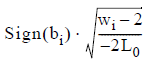where b i is the b- coefficient and w i is the Wald Statistic of the i th independent variable, while L 0 is the initial log likelihood of the model.
If w i <= 2 then Partial R is set to 0. This statistic provides a measure of the relative importance of each variable in the model. It is calculated only when the constant term is included in the model. [SPSS]
• Standardized Coefficient — The estimated standardized coefficient is calculated for each b-coefficient value as: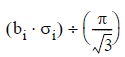where bi is the b-coefficient,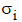is the standard deviation of the i th independent variable, and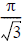is the standard deviation of the standard logistic distribution. This calculation only provides an estimate of the standardized coefficients since it uses a constant value for the logistic distribution without regard to the actual distribution of the dependent variable in the model. [Menard]

## Prediction Success Table

The prediction success table is computed using only probabilities and not estimates based on a threshold value. Using an input table that contains known values for the dependent variable, the sum of the probability values π(x) and 1 – π(x), which correspond to the probability that the predicted value is 1 or 0, respectively, are calculated separately for rows with actual value of 1 and 0. Refer to Logistic Regression Model Evaluation for more information.
• Estimate Response — The entries in the “Estimate Response” column are the sums of the probabilities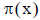that the outcome is 1, summed separately over the observations where the actual outcome is 1 and 0 and then totaled. (Note that this is independent of the threshold value that is used in scoring to determine which probabilities correspond to an estimate of 1 and 0, respectively).
• Estimate Non-Response — The entries in the “Estimate Non-Response” column are the sums of the probabilities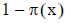that the outcome is 0, summed separately over the observations where the actual outcome is 1 and 0 and then totaled. (Note that this is independent of the threshold value that is used in scoring to determine which probabilities correspond to an estimate of 1 and 0, respectively).
• Actual Total — The entries in this column are the sums of the entries in the Estimate Response and Estimate Non-Response columns, across the rows in the Prediction Success Table. But in fact this turns out to be the number of actual 0s and 1s and total observations in the training data.
• Actual Response — The entries in the “Actual Response” row correspond to the observations in the data where the actual value of the dependent variable is 1.
• Actual Non-Response — The entries in the “Actual Non-Response” row correspond to the observations in the data where the actual value of the dependent variable is 0.
• Estimated Total — The entries in this row are the sums of the entries in the Actual Response and Actual Non-Response rows, down the columns in the Prediction Success Table. This turns out to be the sum of the probabilities of estimated 0s and 1s and total observations in the model.

## Multi-Threshold Success Table

This table provides values similar to those in the prediction success table, but instead of summing probabilities, the estimated values based on a threshold value are summed instead. Rather than just one threshold however, several thresholds ranging from a user specified low to high value are displayed in user specified increments. This allows the user to compare several success scenarios using different threshold values, to aid in the choice of an ideal threshold. Refer to the Model Evaluation section for more information.
• Threshold Probability — This column gives various incremental values of the probability at or above which an observation is to have an estimated value of 1 for the dependent variable. For example, at a threshold of 0.5, a response value of 1 is estimated if the probability predicted by the logistic regression model is greater than or equal to 0.5. The user may request the starting, ending and increment values for these thresholds.
• Actual Response, Estimate Response — This column corresponds to the number of observations for which the model estimated a value of 1 for the dependent variable and the actual value of the dependent variable is 1.
• Actual Response, Estimate Non-Response — This column corresponds to the number of observations for which the model estimated a value of 0 for the dependent variable but the actual value of the dependent variable is 1, a “false negative” error case for the model.
• Actual Non-Response, Estimate Response — This column corresponds to the number of observations for which the model estimated a value of 1 for the dependent variable but the actual value of the dependent variable is 0, a “false positive” error case for the model.
• Actual Non-Response, Estimate Non-Response — This column corresponds to the number of observations for which the model estimated a value of 0 for the dependent variable and the actual value of the dependent variable is 0.

## Cumulative Lift Table

The Cumulative Lift Table demonstrates how effective the model is in estimating the dependent variable. It is produced using deciles based on the probability values. Note that the deciles are labeled such that 1 is the highest decile and 10 is the lowest, based on the probability values calculated by logistic regression. The information in this report, however, is best viewed in the Lift Chart produced as a graph under a Logistic Regression analysis.
• Decile — The deciles in the report are based on the probability values predicted by the model. Note that 1 is the highest decile and 10 is the lowest. That is, decile 1 contains data on the 10% of the observations with the highest estimated probabilities that the dependent variable is 1.
• Count — This column contains the count of observations in the decile.
• Response — This column contains the count of observations in the decile where the actual value of the dependent variable is 1.
• Response (%) — This column contains the percentage of observations in the decile where the actual value of the dependent variable is 1.
• Captured Response (%) — This column contains the percentage of responses in the decile over all the responses in any decile.
• Lift — The lift value is the percentage response in the decile (Pct Response) divided by the expected response, where the expected response is the percentage of response or dependent 1-values over all observations. For example, if 10% of the observations overall have a dependent variable with value 1, and 20% of the observations in decile 1 have a dependent variable with value 1, then the lift value within decile 1 is 2.0, meaning that the model gives a “lift” that is better than chance alone by a factor of two in predicting response values of 1 within this decile.
• Cumulative Response — This is a cumulative measure of Response, from decile 1 to this decile.
• Cumulative Response (%) — This is a cumulative measure of Pct Response, from decile 1 to this decile.
• Cumulative Captured Response (%) — This is a cumulative measure of Pct Captured Response, from decile 1 to this decile.
• Cumulative Lift — This is a cumulative measure of Lift, from decile 1 to this decile.